# KVPY-SX 2018 Chemistry Paper with Solutions

Check out KVPY-SX 2018 Chemistry paper solutions on this page. These step by step solutions are easy to understand. These solutions will help students to increase the speed of solving problems and thus boost their confidence. This helps them to be stress-free during the exam. Students can easily download the PDF format for free to practice paper offline.

### KVPY SX 2018 - Chemistry

Question 1: The amount (in mol) of bromoform (CHBr3) produced when 1.0 mol of acetone reacts completely with 1.0 mol of bromine in the presence of aqueous NaOH is

1. a. 1/3
2. b. 2/3
3. c. 1
4. d. 2

Solution:

The reaction of acetone with Br2 in the presence of NaOH follow as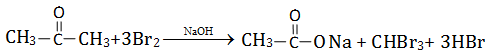Here 3 moles of Br2 reacts with 1 mole acetone, produce 1 mole of CHBr3

So 1 moles of Br2 = 1/3 mole of CHBr3

Hence, the moles of CHBr3 = 1/3 moles

Therefore, the correct option is (a).

Question 2: The following compound can readily be prepared by Williamson ether synthesis by reaction between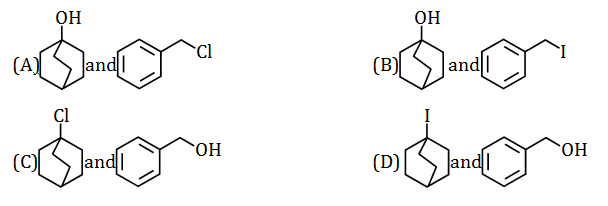Solution:

Williamson ether synthesis ->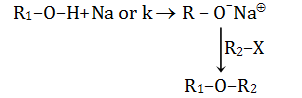Order of reactivity of halide->

R–I > R–Br > R–Cl > R–F (10 > 20 > 30)

Hence, in the following reaction, we can readily prepare the above ether compound.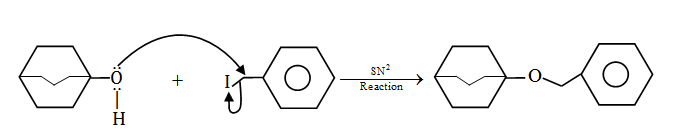Because the stability of carbocation (C+) increases the rate of SN2 decreases.

Therefore, the correct option is (b).

Question 3: X and Y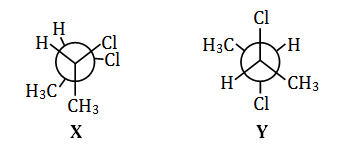are:

1. a. enantiomers
2. b. diastereomers
3. c. constitutional isomers
4. d. conformers

Solution: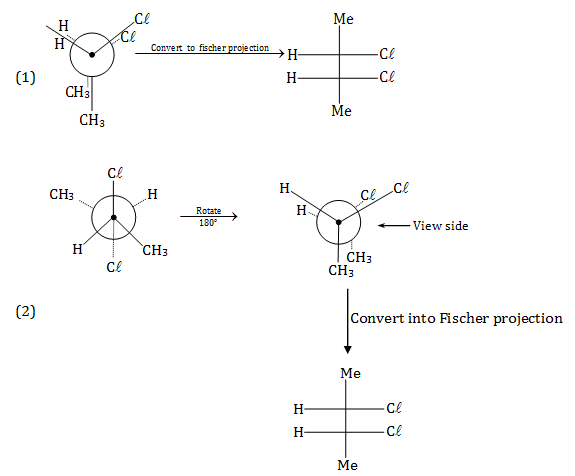(1) and (2) are identical.

Hence, they are conformers.

Therefore, the correct option is (d).

Question 4: The higher stabilities of tert-butyl cation over isopropyl cation, and trans-2-butene over propene, respectively, are due to orbital interactions involving

1. a. σ -> π and σ -> π *
2. b. σ -> vacant p and π -> π *
3. c. σ -> σ * and σ -> π
4. d. σ -> vacant p and σ -> π *

Solution:

Hint:- See the key point and definition of hyperconjugation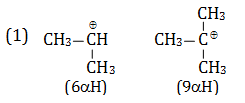Hyperconjugation structure = (Number of αH + 1)

As the α Hydrogen increases the number of Hyperconjugation (σ bond vacant p orbital overlapping) structure increases and stability of carbocation also increases.

Hence, tert-butyl carbocation is more stable compared to isopropyl carbocation.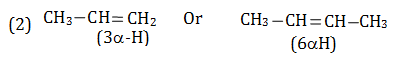Hyperconjugation structure = (Number of αH + 1)

As the α Hydrogen increase in alkene number of Hyperconjugation (σ bond π* Interaction) structure increase and stability of alkene also increase.

Hence, 2 butene is more stable compare to propene.

Therefore, the correct option is (d).

Question 5: Benzaldehyde can be converted to benzyl alcohol in concentrated aqueous NaOH solution using

1. a. acetone
2. b. acetaldehyde
3. c. formic acid
4. d. formaldehyde

Solution:

It is an example of Cannizzaro reaction.

The reaction mechanism follows as

Reactivity order towards Nucleophilic addition -> HCHO >PhCHO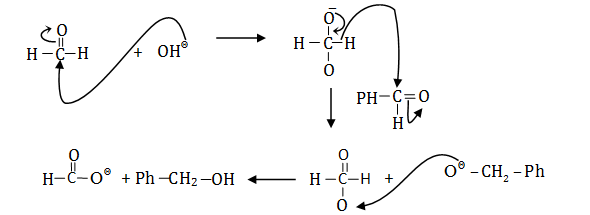Therefore, the correct option is (d).

Question 6: The major product of the following reaction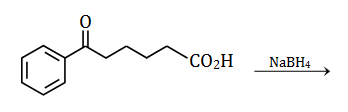is: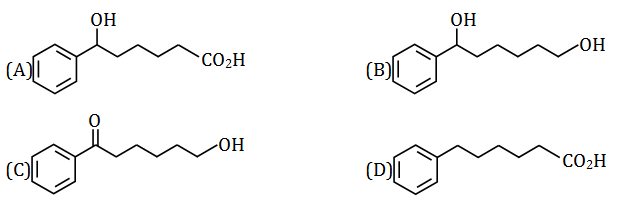Solution:

Solution -> NaBH4 ideal reducing agent for reduction of the carbonyl group to corresponding alcohol without affecting other groups.

The reaction is as follows;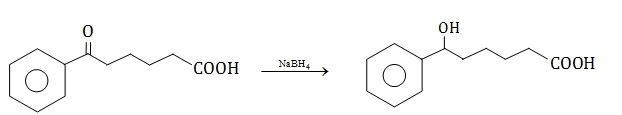Therefore, the correct option is (a).

Question 7: Among the following species, the H–X–H angle (X = B, N or P) follows the order

1. a. PH3< NH3< NH4+< BF3
2. b. NH3< PH3< BF3
3. c. BF3< PH3< NH3
4. d. BF3< NH3< PH3

Solution:

1. PH3-> no hybridisation (Drago’s rule). Bond angle - 930

2.NH3-> sp3 hybridisation (with one lone pair) Bond angle - 1070

Due to lone pair-bond pair repulsion, the bond angle will reduce from 109028’ to 1070.

3.NH4+ -> sp3 hybridisation. Bond angle -109028’

4. BF3-> sp2 hybridisation. Bond angle - 1200

Question 8: The ionic radii of Na+, F, O2–, N3– follow the order

1. a. O2–> F> Na+> N3–
2. b. N3–> Na+> F> O2–
3. c. N3–> O2–> F> Na+
4. d. Na+> F> O2–> N3–

Solution:

Ionic size increases with the addition of electron and size decrease with loss of an electron.

Nitride ion has 10 electrons in contrast with only 7 protons due to the above-given reason. It has the highest inter electronic repulsions. Hence its size or radius is maximum.

Note: For isoelectronic species.

Ionic size is proportional to the negative charge on the anion.

Hence, correct order follow as N3–> O2–> F> Na+

Therefore, the correct option is (c).

Question 9: The oxoacid of phosphorus having the strongest reducing property is:

1. a. H3PO3
2. b. H3PO2
3. c. H3PO4
4. d. H4P2O7

Solution: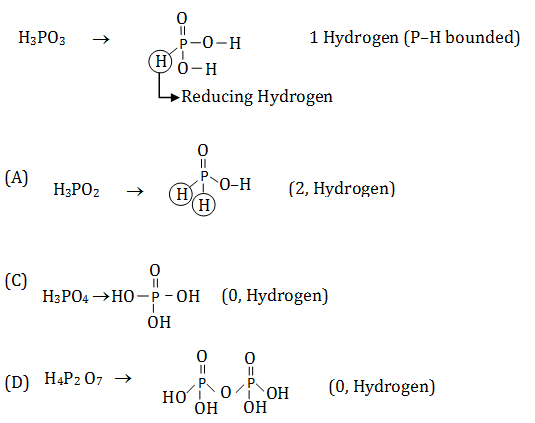Reducing Hydrogen => P–H (Bounded Hydrogen)

-> Hence, in H3PO2 have (2) reducing H due to this it has strongest reducing properties.

Therefore, the correct option is (b).

Question 10: Among C, S and P, the element(s) that produce(s) SO2 on reaction with hot conc. H2SO4 is/are:

1. a. only S
2. b. only C and S
3. c. only S and P
4. d. C, S and P

Solution:

Reaction of C, S and P with H2SO4 follows as

C + H2SO4-> CO2 + H2O + SO2

S + H2SO4-> SO2 + H2O

P + H2SO4-> SO2 + H3PO4 + H2O

=> Hence, here all produced SO2 gas

So, the correct option is (d).

Question 11: The complex that can exhibit linkage isomerism is

1. a. [Co(NH3)5(H2O)]Cl3
2. b. [Co(NH3)5(NO2)]Cl2
3. c. [Co(NH3)5(NO3)](NO3)2
4. d. [Co(NH3)5Cl]SO4

Solution:

Linkage isomerism is the existence of coordination compounds that have the same composition differing from the connectivity of metal to ligand.

=> Here, in case (B) NO2 is a ambidentate ligand So it can bind with metal as N(NO2) and O(ONO).

Hence, compound [Co(NH3)5(NO2)]Cl2 show linkage as follow: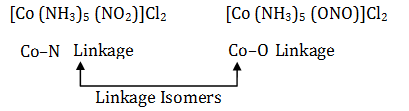Therefore, the correct option is (b).

Question 12: The tendency of X in BX3 (X = F, Cl, OMe, NMe) to form a π bond with boron follows the order

1. a. BCl3< BF3<B(OMe)3< B(NMe2)3
2. b. BF3< BCl3< B(OMe)3< B(NMe2)3
3. c. BCl3<B(NMe2)3< B(OMe)3< BF3
4. d. BCl3< BF3< B (NMe2)3< B(OMe)3

Solution:

In all, highest back bonding in the case of B(NMe2)3 due to less E.N & small size and N have good electron density for back bonding due to +I effect of attached group.

=> E.N increases back bonding decreases.

=> electron density increases back bonding increases.

=> Size increases back bonding decreases.

=> Hence, the overall order of back bonding follow as

B (NMe2)3> B (OMe)3> BF3> BCl3

Therefore, the correct option is (a).

Question 13: Consider the following statements about Langmuir isotherm:

(i) The free gas and adsorbed gas are in dynamic equilibrium

(ii) All adsorption sites are equivalent

(iii) The initially adsorbed layer can act as a substrate for further adsorption

(iv) The ability of a molecule to get adsorbed at a given site is independent of the occupation of neighbouring sites

The correct statements are

1. a. (i), (ii), (iii) and (iv)
2. b. only (i), (ii) and (iv)
3. c. only (i), (iii), and (iv)
4. d. only (i), (ii) and (iii)

Solution:

According to Langmuir isotherm ->

=> ∆H of adsorption of each binding site is same because the surface is homogenous.

=> There is a dynamic equilibrium between free gas and adsorbed gas.

=> The ability of a molecule to get adsorbed at a given site is independent of the occupation of the neighbouring site.

Therefore, the correct option is (b).

Question 14: Among the following, the plot that correctly represents the conductometric titration of 0.05 M H2SO4 with 0.1M NH4OH is: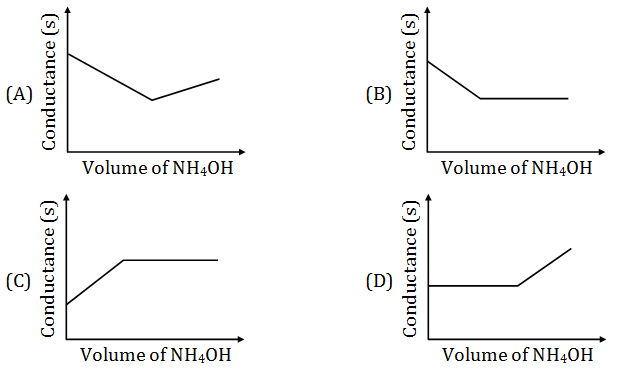Solution:

-> Reaction of H2SO4 with NH4OH follow as.

H2SO4+NH4OH -> (NH4)2SO4 (salt)+H2O

Initial -> H2SO4 is a strong acid so dissociation 100%

Hence, due to the small size of (H+) conductivity is maximum.

=> Upon addition of NH4OH in H2SO4, salt [(NH4)2SO4] will form and small H+ ion is replaced by larger Ion (NH4+) so conductivity decreases.

After salt formation, if we add Excess of NH4OH, there is no change in conductivity because NH4OH is a week base. Hence, the dissociation is very less.

Therefore, the correct option is (b).

Question 15: The correct representation of the wavelength-intensity relationship of ideal blackbody radiation at two different temperatures T1 and T2 is: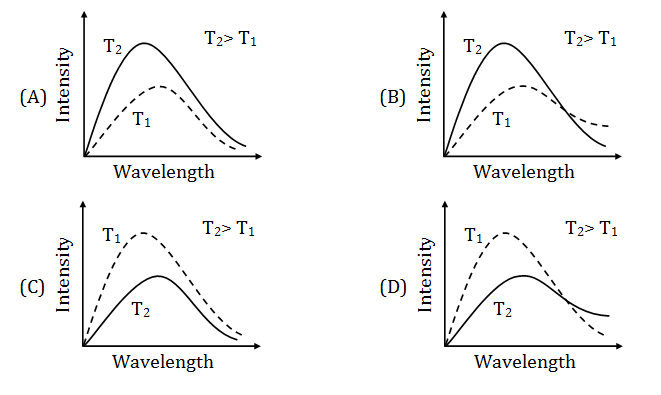Solution:

From Wien's displacement law

λmT =constant

λ proportional to 1/T

=> T increases λ decreases. [At higher temperature λ will be small]

Therefore, the correct option is (a).

Question 16: The pressure (P)-volume (V) isotherm of a van der Waals gas, at the temperature at which it undergoes gas to liquid transition, is correctly represented by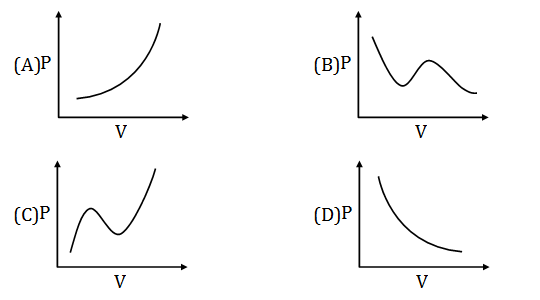Solution: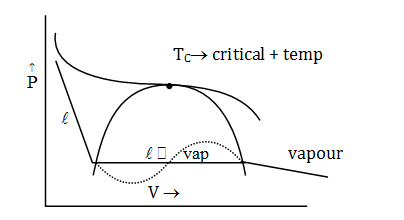Vapour -> liquid (volume decreases )

At critical temperature ( l, in equilibrium)

Therefore, the correct option is (b).

Question 17: A buffer solution can be prepared by mixing equal volumes of

1. a. 0.2 M NH4OH and 0.1 M HCl
2. b. 0.2 M NH4OH and 0.2 M HCl
3. c. 0.2 M NaOH and 0.1 M CH3COOH
4. d. 0.1 M NH4OH and 0.2 M HCl

Solution: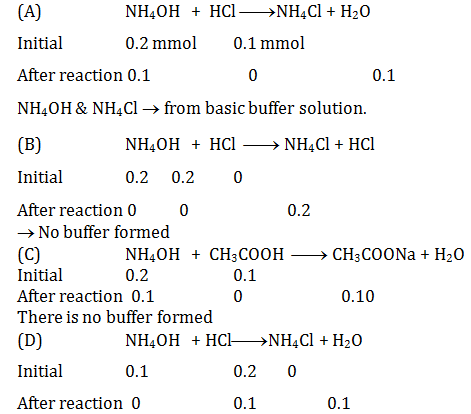-> No buffer formed.

Therefore, the correct option is (a).

Question 18: The plot of total vapour pressure as a function of mole fraction of the components of an ideal solution formed by mixing liquids X and Y is: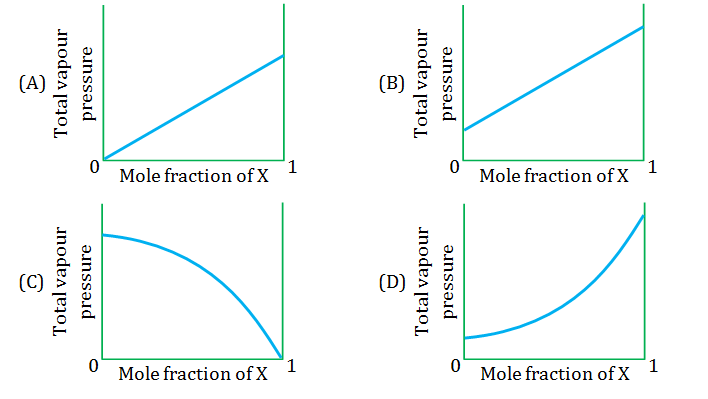Solution:

Since we know that P proportional to x(mole fraction of solute)

For an ideal solution, according to Raoult’s law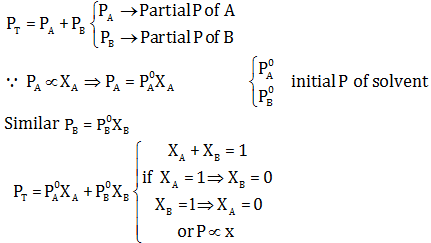Therefore, the correct option is (b).

Question 19: On complete hydrogenation, natural rubber produces

1. a. polyethene
2. b. ethylene-propylene copolymer
3. c. polyvinyl chloride
4. d. polypropylene

Solution:

Natural rubber formed by the polymerization of isoprene and on hydrogenation provides copolymer of ethylene and propylene.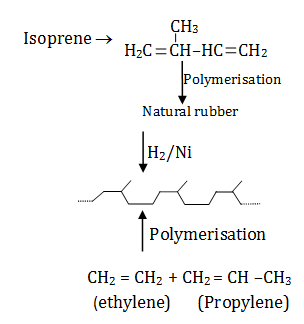Copolymer -> Polymer which is synthesized from more than one species of monomers.

Ex. [A–B–A–B–A–B–A–B --------]n

Therefore, the correct option is (b).

Question 20: The average energy of each hydrogen bond in A-T pair is x kcal mol–1 and that in G-C pair is y kcal mol–1. Assuming that no other interaction exists between the nucleotides, the approximate energy required in kcal mol–1 to split the following double-stranded DNA into two single strands is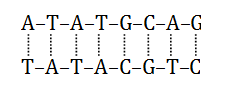[Each dashed line may represent more than one hydrogen bond between the base pairs]

1. a. 10x + 9y
2. b. 5x + 3y
3. c. 15x + 6y
4. d. 5x + 4.5 y

Solution:

Hint -> See the hydrogen bounded structure of A–T (Adenine –Thymine) and –

G–C(Guanine– Cytosine)

Solution

->No OH bond between A–T = 2

Average energy of each H bond in A–T pair = X Kcal mol–1

Total no of A–T pair in structure = 5

Hence, total energy = 2x ×5 = 10 x

-> No of H bond between G–C = 3

Average energy of each H-bond in G–C pair = Y Kcal mol–1

Total no of G–C pair in structure = 3

Hence total energy = 3y×3 = 9y

-> Now, the total energy required to split all double-stranded DNA into two single strands.

=10 x + 9y

Therefore, the correct option is (a).

Question 21: For the electrochemical cell shown below

Pt|H2 (P = 1 atm)|H+(aq., x M)||Cu2+ (aq., 1.0 M)|Cu(s)

The potential is 0.49 V at 298 K. The pH of the solution is closest to

[Given: Standard reduction potential, E0 for Cu2+/Cu is 0.34 V

Gas constant, R is 8.31 J K–1mol–1

Faraday constant, F is 9.65 × 104 J V–1mol–1]

1. a. 1.2
2. b. 8.3
3. c. 2.5
4. d. 3.2

Solution:

Since reduction potential of E0H+/H2 = 0 V & E0Cu+2/Cu = 0.34V

Hence, anode and cathode reaction is as follows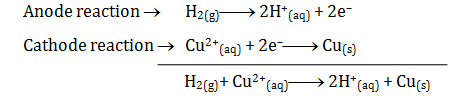E0cell = E0cell - (0.0591/n) log Q {Q = [H+]2/[Cu2+], [H+] = x, [Cu2+] = 1M}

=> 0.49 = 0.34-(0.06/2) log [H+]2

=> 0.15 = +2×(0.06/2) [-log [H+]]/pH

=> pH = 0.15/0.06

{ RT/f = 0.0591, n = 2}

=> pH = 2.5

Therefore, the correct option is (c).

Question 22: Consider the following reversible first-order reaction of X at an initial concentration [X]0. The values of the rate constants are kf = 2s–1 and kb = 1s–1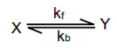A plot of concentration of X and Y as function of time is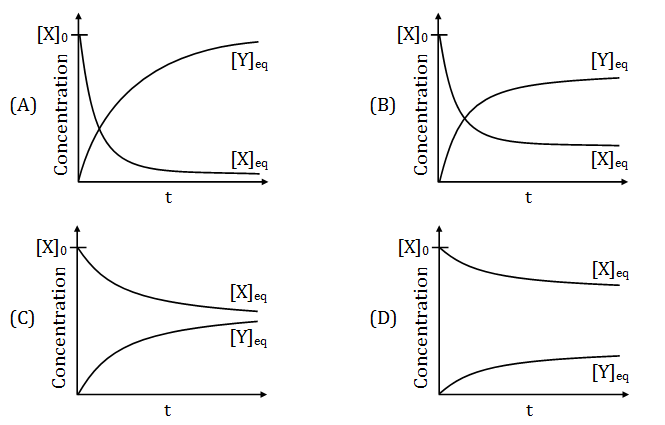Solution: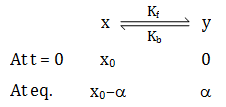Keq = Kf/Kb = α/x0- α = 2

{ Keq = Kf/Kb =2/1 = 2}

Kf -> rate constant for forward reaction

Kb -> rate constant for backward reaction

=> α = 2x0 – 2α

=> 3α = 2x0

=> α = 2/3 x0

At equilibrium

[x] = x0/3

[y] = 2/3x0

Hence, here concentration ‘y’increases equal to concentration ‘x’decreases.

So, graph (B) best represents this.

Therefore, the correct option is (b).

Question 23: Nitroglycerine (MW = 227.1) detonates according to the following equation:

2C3H5(NO3)3(l) -> 3N2(g) + ½O2(g) + 6CO2(g) + 5H2O(g)

The standard molar enthalpies of formation of ΔHf0 for all the compounds are given below:

ΔHf0 [C3H5(NO3)3] = –364 kJ/mol

ΔHf0 [CO2(g)] = –393.5 kJ/mol

ΔHf0 [H2O(g)] = –241.8 kJ/mol

ΔHf0 [N2(g)] = 0 kJ/mol

ΔHf0 [O2(g)] = 0 kJ/mol

The enthalpy change when 10 g of nitroglycerine is determined is

1. a. –100.5 kJ
2. b. –62.5 kJ
3. c. –80.3 kJ
4. d. –74.9 kJ

Solution:

Reaction

2C3H5(NO3)3(l) -> 3N2(g) + ½O2(g) + 6CO2(g) + 5H2O(g)

We know that ΔHf0 = (ΔHf0)P- (ΔHf0)Reactant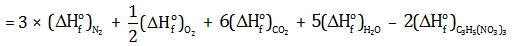= 0 + 0 + 6 × (–393.5) + 5 × (–241.8) – 2 × (–364)

ΔHf0 = –2842 kJ

Therefore for one mole nitroglycerine ΔHf0 = -2812/2= –1421 kJ mole–1

Wt of nitroglycerine = 10 g

Mw of nitroglycerine = 227

Moles of nitroglycerine = 10/227 moles

Hence, (ΔHf0 ) for 10/227 moles = 1421 × 10/227.1 = 62.5 kJ

Therefore, the correct option is (b).

Question 24: The heating of (NH4)2Cr2O7 produces another chromium compound along with N2 gas. The change of the oxidation state of Cr in the reaction is

1. a. +6 to +2
2. b. +7 to +4
3. c. +8 to +4
4. d. +6 to +3

Solution:

Heating reaction of (NH4)2Cr2O7 follow as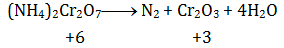Hence, change in the oxidation state of Cr -> +6 to +3

Therefore, the correct option is (d).

Question 25: The complex having the highest spin-only magnetic moment is

1. a. [Fe(CN)6]3–
2. b. [Fe(H2O)6]2+
3. c. [MnF6]4–
4. d. [NiCl4]2–

Solution:

(1)[Fe(CN)6]3–

Fe3+ -> d5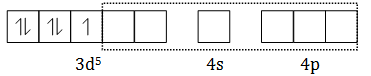(d2sp3-> Hybridisation)

CN- -> Strong field ligand, hence, pairing occur.

Unpaired electron = 1

MM = √(n(n+2)) = 1.732 BM

(2)[Fe(H2O)6]2+

Fe2+-> d6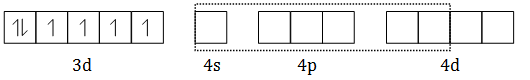(sp3d2-> Hybridisation)

H2O->Weak field ligand, hence, the pairing does not occur.

Unpaired electron = 4

MM = 4.90 BM

(3)[MnF6]4–

Mn2+-> d5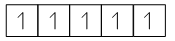F-> Weak field ligand

Hence, pairing not occur

Unpaired electron = 5

MM = 5.92 BM

(4)[NiCl4]2–

Cl-> Weak field ligand

Hence, paring not occur

Ni2+-> d8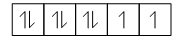MM = 2.83 BM

Therefore, the correct option is (c).

Question 26: Among Ce(4f1 5d1 6s2), Nd(4f4 6s2), Eu(4f7 6s2) and Dy(4f10 6s2), the elements having highest and lowest 3rd ionization energies, respectively, are

1. a. Nd and Ce
2. b. Eu and Ce
3. c. Eu and Dy
4. d. Dy and Nd

Solution:

Ionisation energy -> Minimum amount of energy required to remove the most loosely bonded electron of an isolated neutral gaseous atoms or molecules.

 1 Ce Ce2+ 4f15d16s2 4f2 • Least stable • Hence, need least amount of energy to remove the electron 2 Nd Nd2+ 4f46s2 4f4 3 Eu Eu2+ 4f76s2 4f7 • Half field -> most stable, hence need more energy to remove the electron 4 Dy Dy2+ 4f106s2 4f10

Therefore, the correct option is (B).

Question 27: The major product of the following reaction sequence is: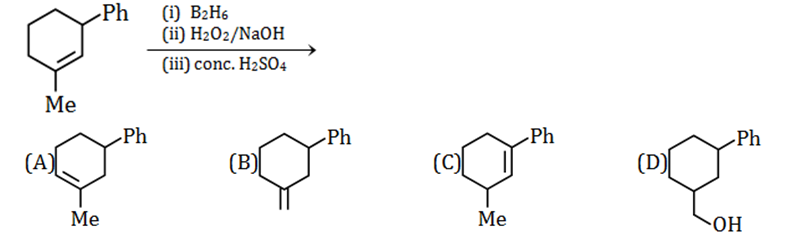Solution: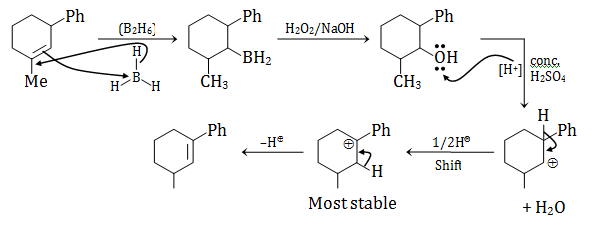Therefore, the correct option is (c).

Question 28: Among the following reactions, a mixture of diastereomers is produced from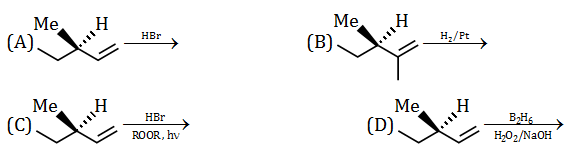Solution: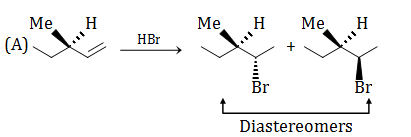Diastereomers-> Stereoisomers that are not mirror images, different compounds with different physical properties.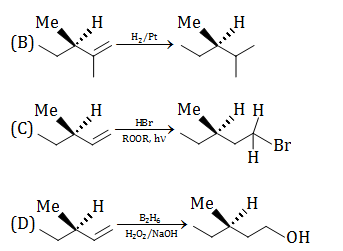Therefore, the correct option is (a).

Question 29: Reaction of phenol with NaOH followed by heating with CO2 under high pressure, and subsequent acidification gives compound X as the major product, which can be purified by steam distillation. When reacted with acetic anhydride in the presence of a trace amount of conc. H2SO4, compound X produces Y as the major product. Compound Y is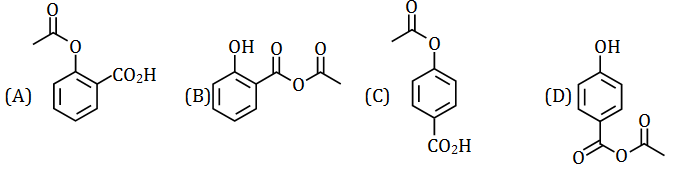Solution: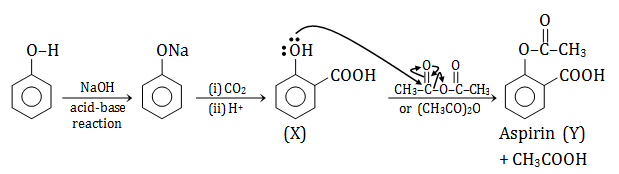Therefore, the correct option is (a).

Question 30: A tetrapeptide is made of naturally occurring alanine, serine, glycine and valine. If the C-terminal amino acid is alanine and the N-terminal amino acid is chiral, the number of possible sequences of the tetrapeptide is

1. a. 12
2. b. 8
3. c. 6
4. d. 4

Solution: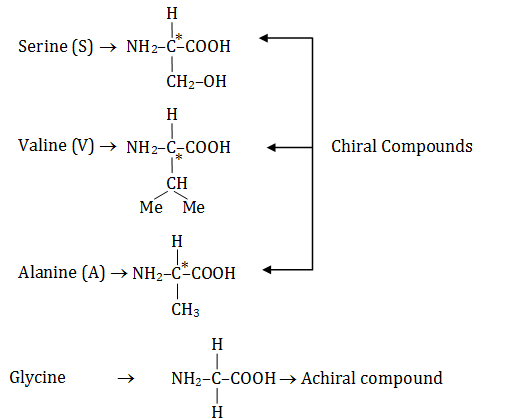Possible sequence:

Ser – Val – Gly – Ala

Ser – Gly – Val – Ala

Val – Ser – Gly – Ala

Val – Gly – Ser – Ala

Total 4.

Therefore, the correct option is (d).

### KVPY SX 2018 Chemistry Paper with Solutions